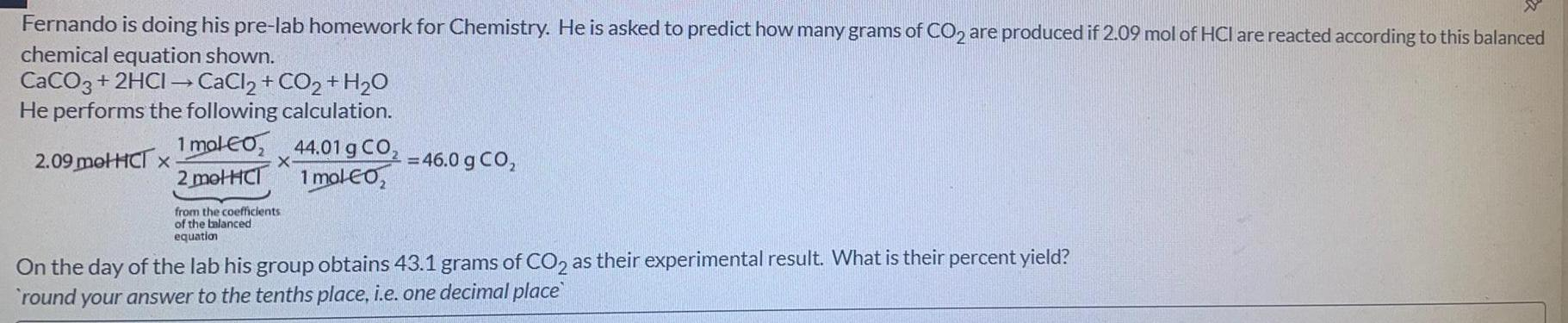Question:

# Fernando is doing his pre-lab homework for Chemistry. He is

Last updated: 8/9/2022Fernando is doing his pre-lab homework for Chemistry. He is asked to predict how many grams of CO2 are produced if 2.09 mol of HCI are reacted according to this balanced chemical equation shown. CaCO3 + 2HCI → CaCl2 + CO2 + H2₂O He performs the following calculation. 1 mol CO₂ 2 metHCI 2.09 metHCT x X from the coefficients of the balanced equation 44.01 g CO₂ 1 mol CO₂ = 46.0 g CO₂ On the day of the lab his group obtains 43.1 grams of CO2 as their experimental result. What is their percent yield? round your answer to the tenths place, i.e. one decimal place